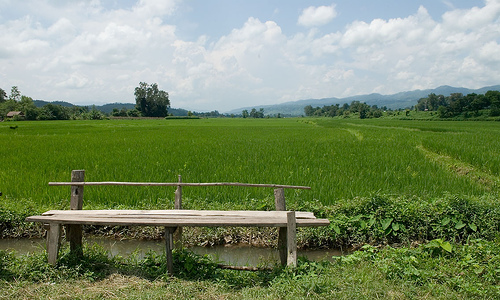# Hsipaw

﻿
Hsipaw

Infobox Settlement
official_name = Hsipaw
other_name = Thibaw
native_name = _my. သီပေါ
nickname =
settlement_type =
motto =imagesize = 300px
image_caption = Hsipaw

flag_size =
image_

seal_size =
image_shield =
shield_size =
image_blank_emblem =
blank_emblem_type =
blank_emblem_size =

mapsize =
map_caption =

mapsize1 =
map_caption1 =
image_dot_

dot_mapsize =
dot_map_caption =
dot_x = |dot_y =
pushpin_pushpin_label_position =bottom
pushpin_map_caption =Location in Burma
subdivision_type = Country
subdivision_name = Burma
subdivision_name1 = Shan State
subdivision_type2 =
subdivision_name2 =
subdivision_type3 =
subdivision_name3 =
subdivision_type4 =
subdivision_name4 =
government_footnotes =
government_type =
established_title =
established_date =
established_title2 =
established_date2 =
established_title3 =
established_date3 =
area_magnitude =
unit_pref =Imperial
area_footnotes =
area_total_km2 =
area_land_km2 =
area_water_km2 =
area_total_sq_mi =
area_land_sq_mi =
area_water_sq_mi =
area_water_percent =
area_urban_km2 =
area_urban_sq_mi =
area_metro_km2 =
area_metro_sq_mi =
area_blank1_title =
area_blank1_km2 =
area_blank1_sq_mi =
population_as_of =2005
population_footnotes =
population_note =
population_total =
population_density_km2 =
population_density_sq_mi =
population_metro =
population_density_metro_km2 =
population_density_metro_sq_mi =
population_urban =
population_density_urban_km2 =
population_density_urban_sq_mi =
population_blank1_title =Ethnicities
population_blank1 =
population_blank2_title =Religions
population_blank2 =Buddhism
population_density_blank1_km2 =
population_density_blank1_sq_mi =
timezone =
utc_offset =
timezone_DST =
utc_offset_DST =
latd=22 |latm=37 |lats= |latNS=N
longd=97 |longm=17 |longs= |longEW=E
elevation_footnotes =
elevation_m =
elevation_ft =
postal_code_type =
postal_code =
area_code =
blank_name =
blank_info =
blank1_name =
blank1_info =
website =
footnotes =

Hsipaw ( _my. သီပေါ; also known as Thibaw), is a town in Shan State, Myanmar. It is 200 km (124 mi) northeast of Mandalay.

In the 1890s Christian missionaries laboured in Thibaw. One missionary martyr of Thibaw is commemorated in the books The missionary martyr of Thibaw: A brief record of the life and consecrated missionary labours of Charles William Lambert in upper Burmah. His death is commemorated by a headstone in Charlton Cemetery, London SE4.

Wikimedia Foundation. 2010.